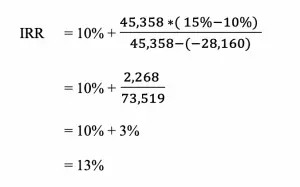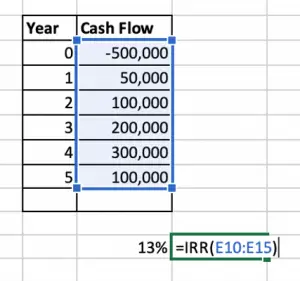# Internal Rate of Return

Internal rate of return (IRR) is the tool that the company uses to measure the performance of the investments’ project. It is the discounted rate that makes the Net Present Value of the project equal to zero. When we use the IRR to discount future cash flow (both inflow and outflow) of a project, the total will be zero. It means that the present value of future cash inflow equal to the present value of cash outflow by using IRR.

We use IRR to evaluate the project before making any investment decisions. It is very useful when there are many opportunities available and limited funds. So the company tries to make precise decisions by investing in high profitable project.

In some companies, management already approves the minimum rate, hurdle rate, so new investments must be equal or higher than this rate. Any project with a lower rate should not be put into consideration.

## IRR Formula

$Internal\ Rate\ of\ Return = LR + {LNPV\ * (HR – LR) \over (LNPV-HNPV)}$

LR      =   Lower discount rate

HR      =   Higher discount rate

HNPV   =   Higher Net Present Value

LNPV   =   Lower Net Present Value

## Example

Company A is considering the new investment with cash flow as following

Year Cash Flow
0 (500,000)
1 50,000
2 100,000
3 200,000
4 300,000
5 100,000

The company has the cost of a capital of 10%. Please calculate IRR and give recommendations on Company A if they should make this investment.

1. Let select the two rate and calculate the NPV

We assume:

• LR is 10%
• HR is 15%
1. Calculate the NPV of cash flow using these two rates
Year Cash Flow 10% NPV@ 10% 15% NPV@ 15%
0 (500,000) 1          (500,000) 1 (500,000)
1 50,000        0.909               45,455       0.870           43,478
2 100,000        0.826               82,645       0.756           75,614
3 200,000        0.751             150,263       0.658         131,503
4 300,000        0.683             204,904       0.572         171,526
5 100,000        0.621               62,092       0.497           49,718
45,358          (28,160)
NPV 1         NPV 2
1. Calculate IRR1. Interpret

It means that the investment will generate return 13% per year compound. It is higher the cost of capital, so the company should consider this project.

Note: IRR is the discounted rate which makes NPV of the project to zero. Let reverse the calculation to check whether it is zero or not.

 Year Cash Flow 13% NPV@ 13% 0 -500,000 1 (500,000) 1 50,000 0.885 44,248 2 100,000 0.783 78,315 3 200,000 0.693 138,610 4 300,000 0.613 183,996 5 100,000 0.543 54,276 (556)

It is not precisely zero due to the rounding error from our manual calculation.

## Calculate Internal Rate of Return using Excel

Using excel formula to calculate IRR is very straight forward, we just using the excel formula (IRR) and select the cash flow from all periods. Then, Excel will do the work for us.The result can be slightly different from the manual.

## What are the Benefits of Internal Rate of Return?

Benefit of IRR
Easy to understand IRR is very easy to understand. Company is looking for a higher rate.
Easy to compare projects The company may have many opportunities to invest and it hard to select the best option. With IRR, it is easier to compare between them. Moreover, it also enables us to compare the return and cost of capital to ensure profitability.
Consider the time value of money This method considering the time value of money by discount all future cash flow to make the calculation. It makes the process of calculation more accurate.